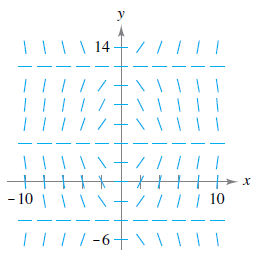Chapter 6.1, Problem 55E

Chapter
Section
Textbook Problem

Slope Field In Exercises 53–56, a differential equation and its slope field are given. Complete the table by determining the slopes (if possible) in the slope field at the given points. x - 4 - 2 0 2 4 8 y 2 0 4 4 6 8 d y d x d y d x = x   cos   π y 8To determine

To Calculate: The slopes (if possible) in the slope field at the points provided.

Explanation

Given:

The differential equation: dydx=xcos(πy8)

Formula Used:

dydx=xcos(πy8)

Calculation:

It is given that the differential equation is:

dydx=xcos(πy8)

At the point (4,2) the slope is: dydx=(4)cos(π.28)=4cos(π4)=4.12=22

At the point (2,0) the slope is: dydx=(2)cos(π.08)=2cos(0)=2.1=2

At the point (0,4) the slope is: dydx=0.cos(π.48)=0.cos(π2)=0

At the point (2,4) the slope is: dydx=2

Still sussing out bartleby?

Check out a sample textbook solution.

See a sample solution

The Solution to Your Study Problems

Bartleby provides explanations to thousands of textbook problems written by our experts, many with advanced degrees!

Get Started

Find more solutions based on key concepts